##### Actions

A square matrix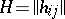of order, with entries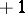or, such that the equation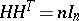(*)

holds, where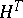is the transposed matrix ofand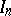is the unit matrix of order. Equality (*) is equivalent to saying that any two rows ofare orthogonal. Hadamard matrices have been named after J. Hadamard who showed  that the determinant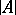of a matrix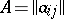of order, with complex entries, satisfies the Hadamard inequality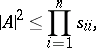where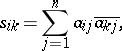and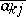is the element conjugate to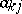(cf. Hadamard theorem on determinants). In particular, if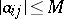, then. It follows that a Hadamard matrix is a square matrix consisting of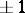's with maximal absolute valueof the determinant. The properties of Hadamard matrices are: 1)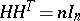implies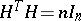and vice versa; 2) transposition of rows or columns and multiplication of the elements of an arbitrary row or column by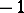again yields a Hadamard matrix; 3) the tensor product of two Hadamard matrices is also a Hadamard matrix, of order equal to the product of the orders of the factors. In other words, ifand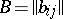are Hadamard matrices of ordersandrespectively, then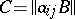is a Hadamard matrix of order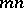. A Hadamard matrix with its first row and first column consisting only ofterms is said to be normalized. The order of a Hadamard matrix is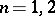or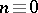(). The normalized Hadamard matrices of orders 1 and 2 are:The existence of a Hadamard matrix has been demonstrated for several classes of values of(see, for example, , ). At the time of writing (the 1980s), it has not yet been proved that a Hadamard matrix exists for any(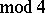). For methods of constructing Hadamard matrices see . Hadamard matrices are used in the construction of certain types of block designs  and codes  (cf. Block design; Code). A Hadamard matrix of order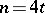is equivalent to a-design.

A generalized Hadamard matrix is a square matrix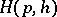of order, with as entries-th roots of unity, which satisfies the equality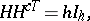whereis the conjugate transpose of the matrixandis the unit matrix of order. Generalized Hadamard matrices have properties analogous to 1) and 3) (cf. ).